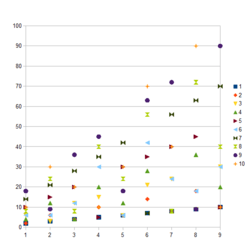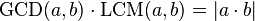# Least common multiple facts for kids

Kids Encyclopedia FactsLCMs of numbers 1 through 10 with numbers 2 through 10. Line labels = first number. X axis = second number minus 1. Y axis = LCM of the two numbers.

The least common multiple of two integers is the smallest positive integer between all the multiples of both. It is usually denoted by LCM(a, b). Likewise, the LCM of more than two integers is the smallest positive integer that is divisible by each of them.

The LCM is known in elementary arithmetic as the "least common denominator" (LCD) that must be calculated before fractions can be added, subtracted or compared.

## Overview

A multiple of a number is the product of that number and an integer. For example, 10 is a multiple of 5 because 5 × 2 = 10, so 10 is divisible by 5 and 2. Because 10 is the smallest positive integer that is divisible by both 5 and 2, it is the least common multiple of 5 and 2. By the same principle, 10 is the least common multiple of −5 and 2 as well.

## Relation with the greatest common divisor

It is known that:$\operatorname{GCD}(a,b) \cdot \operatorname{LCM}(a,b) = |a \cdot b|$

This formula is often used to compute the LCD, once known the GCD of a and b.

## Related pagesLeast common multiple Facts for Kids. Kiddle Encyclopedia.